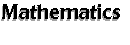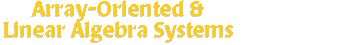[an error occurred while processing this directive] With software in this section, you can handle calculations of array-oriented (vector, matrix) data easily without worrying about the detailed programming techniques. Many of the software here contain classical numerical routines like Linpack, Eispack, Lapack, Blas, from Netlib, so that you have access to these powerful numerical linear algebra routines within a single package. Also, many software here have gone even further to include lots of capabilities like data processing & visualization, nonlinear system, application development, etc. Search SAL: Array-Oriented & Linear Algebra Systems   (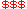Commercial,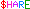Shareware,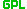GPL) Classic MATLAB -- freeware (old) MATLAB with linear algebra routines. Euler -- numerical matrix language. GAUSS-- a data analysis environment based on the GAUSS matrix language. Gnatlab-- a tool for fast matrix manipulation and calculation. HCL-- a C++ class library to implement vectors and linear operators. IDL-- data analysis, visualization and application development. idl2mat -- enable communication from IDL to Matlab. magicsqr-- a magic squares generator. MATCALC -- easy-to-use matrix calculation package. MATCOM-- MATLAB to C++ translator and C++ matrix class library. MATLAB-- interactive numeric computation and visualization.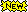Matrix Math-- a software to quickly and easily compute functions of matrices. Matwrap -- a wrapper generator for matrix languages. MathXplorer/JS-- a distributed math server providing matlab-compatible numerical calculation. MathXplorer/T -- provides matrix-based numerical analysis capabilities within Tcl. matlisp -- a package for Common Lisp for handling matrics computation. Matrix-- a Matlab compatible C++ matrix class library. MatPy-- numerical linear algebra and plotting with Python. Matvec -- object-oriented matrix-vector computations.MaTX -- a high-performance programming language for numerical and symbolic computation. NumPy -- add powerful multi-dimensional array objects to Python. GMatH-- mathematical computing interface for GNOME. Octave-- numerical computations mostly compatible with MATLAB.GOctave-- a GNOME-based frontend for GNU Octave. Ox -- object-oriented matrix language similar to C/C++. PDL (or PerlDL) -- turn perl into an array-oriented, numerical language.PLab -- a simple interface for parallelizing Matlab computations. RLaB-- matrix oriented, interactive programming environment. KLab -- a plotter and GUI for RLaB. Scilab -- matrix-based scientific software resembling MATLAB. Simulink-- modeling, analyzing, and simulating dynamic nonlinear systems, under MATLAB. TELA-- large-scale numerical simulations and data processing. uMatrix -- a very simple program for several matrix operations. Xi -- interactive C-like programming language with vector extensions. Yorick -- fast interpreted languages for numeric computing, analysis and processing. Also Check Out: Aladdin -- computational toolkit for interactive matrix and finite element analysis. ANA -- extensive, interactive data and image processing software package and language. Algae-- a high-level interpreted language for numerical analysis. Clif-- a C-like interpreter framework. Isaac -- scientific calculator and programming language. J-- high level language emphasized on functional programming and array processing. Multipack-- math Fortran routines linked to Python. Newmat -- a C++ matrix library. TMath -- a Tcl interface to Matlab and Mathematica. *X SC Interval Arithmetic SW -- allowing numerical analysis using interval arithmetic to control numerical errors. Other Useful Links Matrix Market: A visual repository of test data for use in comparative studies of algorithms for numerical linear algebra, featuring nearly 500 sparse matrices from a variety of applications, as well as matrix generation tools and services. http://math.nist.gov/MatrixMarket/Computer Algebra Systems   |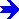Statistics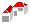SAL Home   |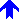Mathematics